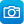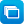# Search results

Year level
Resource type
Learning area

## Refine by topic

Main topic Specific topic Related topic
Listed under:  ArraysThese seven learning activities, which focus on 'open-ended tasks' using a variety of tools (software) and devices (hardware), illustrate the ways in which content, pedagogy and technology can be successfully and effectively integrated in order to promote learning. In the activities, teachers use investigations in order ...### Mental computation: basic facts - multiplication and division

This is a teacher resource that is part of a wider series; it develops a structured strategies-based approach to mental computation of single-digit multiplication and related division facts at lower primary level. It provides 22 engaging classroom activities that will stimulate students to use appropriate mental-computation ...### Multiplication and division

Use these resources to develop your students' understanding and use of efficient strategies for multiplication and solving problems in authentic situations. Develop strategies for a sound basis of skip counting and other examples of repeated addition. Investigate division by sharing quantities (partition) then by repeated ...### The multiplier: make your own hard multiplications

Solve multiplications such as 84x93. Use a partitioning tool to help solve your own multiplications. Learn strategies to do complex arithmetic in your head. Split a sum into parts that are easy to work with, use simple times tables, then solve the original calculation. This learning object is one in a series of five objects.### The multiplier: generate easy multiplications

Solve multiplications such as 9x88. Use a partitioning tool to help solve randomly generated multiplications. Learn strategies to do complex arithmetic in your head. Split a multiplication into parts that are easy to work with, use simple times tables, then solve the original calculation. This learning object is one in ...### The multiplier: generate hard multiplications

Solve multiplications such as 67x88. Use a partitioning tool to help solve randomly generated multiplications. Learn strategies to do complex arithmetic in your head. Split a multiplication into parts that are easy to work with, use simple times tables, then solve the original calculation. This learning object is one in ...### The multiplier: go figure

This tutorial is suitable for use with a screen reader. It explains strategies for solving complex multiplications in your head such as 22x38. Work through sample questions and instructions explaining how to use partitioning techniques. Solve multiplications by breaking them up into parts that are easy to work with, use ...### Pobble arrays: find factors: assessment

Test your understanding of arrays by arranging pobbles into equal columns and rows. Look at the given number of pobbles and enter the number of gates and rows. For example, decide how many equal rows of pobbles are needed to fit 24 of them through four gates. The object starts with a practice task, which provides text and ...### Pobble arrays: make multiples: assessment

Test your understanding of arrays by arranging pobbles into equal columns and rows. For example, start with 19 pobbles. Decide whether the number can be divided into an equal number of rows. If not, add or subtract pobbles to make a number that will work. The object starts with a practice task, which provides text and animation ...### Packing jelly beans - mathematics activities

The photograph shows a box containing jelly beans in an array. Looking closely, we see each compartment in this box contains six jelly beans of the same colour. They are arranged in two layers of three beans. There is a different flavour in each compartment. Teachers are encouraged to scan all the ideas suggested here as ...### Exploring division and multiplication

This collection of 17 digital curriculum resources contains activities to consolidate and extend students' problem-solving skills in the areas of multiplication and division. The first category, arrays, allows students to visualise problems while consolidating the idea that multiplication involves repeated groups of objects. ...### Using 'Multiplying and dividing with arrays' - Teacher idea

This Teacher idea includes a teacher’s reflection on teaching R11453 'Multiplying and dividing with arrays - unit of work' with her mixed ability class. The unit explores the concepts of multiplication and division, integrating digital and non-digital resources, and offers students a range of visual, written and mental ...### Multiplying and dividing with arrays - unit of work

This unit explores the concepts of multiplication and division and offers students strategies to perform these operations.### Introducing arrays - unit of work

This unit explores how arrays can be used to perform the operations of multiplication and division.### The number partner: go figure

This tutorial is suitable for use with a screen reader. It explains strategies for breaking up numbers into pairs of smaller numbers, eg 15 = 11 + 4. Work through examples of whole number pairs and sample questions. Apply these principles to solve additions or subtractions.### The array

Use an array-building tool to help solve multiplications. Explore strategies to break up multiplications. Create and solve easy multiplications such as 9x3. Examine relationships between rows, columns and areas in arrays.### The array: go figure

This tutorial is suitable for use with a screen reader. It explains strategies for solving simple multiplications in your head such as 6x4. Work through sample questions and instructions explaining how to break up numbers into their factors. Solve multiplications by using arrays to break them up into rows and columns, then ...### The difference bar: make your own hard subtractions

Learn how to split up numbers in your head. Use a linear partitioning tool to help find the difference between pairs of two-digit numbers such as 27 and 86. Choose your own pairs of numbers. Split the numbers into parts that are easy to work with, work out each part and then solve the original calculation. This learning ...### The difference bar: generate hard subtractions

Learn how to split up numbers in your head. Use a linear partitioning tool to help find the difference between pairs of two-digit numbers such as 25 and 44. In most of these examples, the difference is greater than ten. Split the numbers into parts that are easy to work with, work out each part and then solve the original ...### The difference bar: go figure

This tutorial is suitable for use with a screen reader. It explains how to split up numbers in your head when finding the difference between two numbers such as 26 and 73. Work through sample questions and instructions explaining how to use linear partitioning techniques. Find the difference between pairs of numbers. Split ...